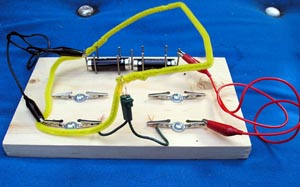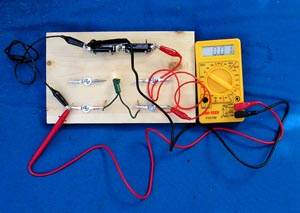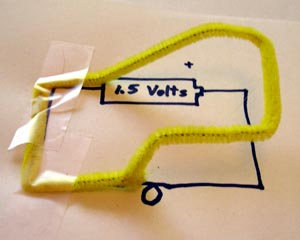Wire Model of Voltage

I've got it wiredA pipecleaner model of the voltage around a circuit.

Introduction

Voltage around a circuit can be modeled by using the height of a bendable wire.

Material

Chenille stems (AKA Pipe cleaners)

A circuit

We use the bulbs cut from strings of 50 Christmas tree bulbs.

A voltmeter

Assembly

Wire together a simple circuit, start by attaching one battery to one bulb.

It is easiest to use a breadboard to wire up the circuit.

To Do and Notice

In your circuit choose a reference point. The black lead to the COM terminal of your voltmeter will remain attached to this reference point for every measurement in this activity.

In a one-battery circuit it is usual to choose the negative terminal of the battery as the reference or common point.

We are going to make a model of the voltage at points around the circuit using the height of wires above the board to represent the voltage relative to the reference point.

Start by placing a wire on the board at the negative terminal of the battery, this wire is at height 0 representing 0 voltage difference with respect to the reference point.

Measure the voltage (see meter voltage) at the side of the bulb connected to the negative side of the battery. It is also at zero volts so the wire remains at 0 height from the negative terminal of the battery to the bulb.The black lead from the meter is the reference, the red lead measures voltage.

Measure the voltage between the reference point and the side of the bulb connected to the positive terminal of the battery. It should be + 1.5 volts. Bend the wire up so that it is 1.5 inches (4 cm) above the surface.

Measure the voltage between the reference point and the positive terminal of the battery. It will be 1.5 volts. Keep the wire at 1.5 inches (4 cm)between the bulb and the battery.

Drop the wire from 1.5 inches (4 cm) to 0 height across the battery to complete the circuit.

You have now made a model for the voltage around a circuit representing voltage as height. See the image at the top of this page.

Notice that the voltage goes up in the batteries, and that it drops across the bulb.

What's Going On?

Voltage is the energy in joules per coulomb of electric charge. Thus a positive charge of q = 1 coulomb at a voltage of V =1.5 volts has an electric potential energy, U = 1.5 joules.

U = qV

Gravitational potential energy is proportional to height. A mass of m = 1 kg at a height of h = 15 cm above a reference level on earth where the acceleration of gravity is g = 10 m/s^2 has a gravitational potential energy U = 1.5 joules

U = mgh

So height can be used to model voltage.

So What?

A positive electric charge going around a circuit has electrical potential energy proportional to the voltage. It has high energy at the positive terminal of the battery and low energy at the negative terminal of the battery. Starting at the negative terminal of the battery it moves through a wire to the bulb losing very little of its energy (height). When it passes through the bulb the potential energy of the positive charge is converted into thermal energy in the filament of the bulb. The voltage and so the height drops linearly through the bulb to zero height. Then the charge flows through the wire to the negative terminal of the battery. Inside the battery the electric charge is given electric potential energy again. It is at 1.5 volts as it exits the positive terminals. Chemical energy in the battery provides the increase in electrical potential energy to the charge.

Now in this circuit outside of the battery it is actually the negative charges that move. Since the electric potential energy is proportional to the charge and the charge is negative then the wires representing the potential energy of the negative charges should be inverted. The charges start out at 0 height at the negative terminal and drop to -1.5 inches (- 4 cm) at the positive terminal of the battery. This added complexity is one reason to use the model of electric circuits in which positive charges do the moving.

Inside the battery things get complicated, positive charges move one way while negative charges move the other.

Going further

Try other circuits. Use two batteries, use two bulbs. Hook up the bulbs in series, hook up the bulbs in parallel. Hook up the batteries in parallel.

What happens if you choose your reference point at the positive terminal of the battery? (You end up with negative voltages with respect to this reference and negative heights.)

You can also make models of the voltage around a circuit drawing.The height of a wire models the voltage around a circuit.

 Scientific Explorations with Paul Doherty © 2005 19 Sep 2005